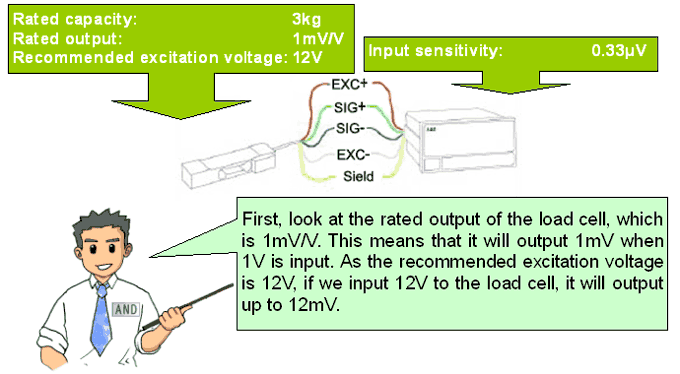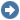# Products8. How should we select a load cell?

The common procedure is as follows:Below is an example of how to connect an indicator and a load cell.

Suppose we prepared a load cell whose capacity is larger than 3.6kg to measure 3kg. We would like to display the results using an indicator, but what kind of indicator should we use? The specifications of the load cell and the indicator are as follows:Let’s presume that the load cell will output approx. 12mV when 3kg is loaded,Suppose the indicator displays the weight in units of 0.1g between 0 and 3kg. This 0.1g is called the minimum scale division. As 3kg is divided by 30000 divisions (0.1g), the resolution is said to be. Per 0.1g, the output will be:The input sensitivity of the indicator is 0.33μV.As this value is smaller than 0.4μV, we can use this indicator. If the input sensitivity is larger than 0.4μV, the indicator cannot display the weight in units of 0.1g.BACK NEXTLoad Cells Q&A TOP

Page Top